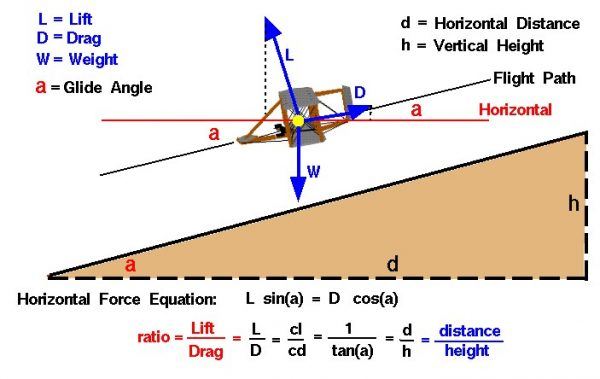# Lift to Drag Ratio## Four Forces

There are four forces that act on an aircraft in flight: lift, weight, thrust, and drag. Forces are vector quantities having both a magnitude and a direction. The motion of the aircraft through the air depends on the relative magnitude and direction of the various forces. The weight of an airplane is determined by the size and materials used in the airplane’s construction and on the payload and fuel that the airplane carries. The weight is always directed towards the center of the earth. The thrust is determined by the size and type of propulsion system used on the airplane and on the throttle setting selected by the pilot. Thrust is normally directed forward along the center-line of the aircraft. Lift and drag are aerodynamic forces that depend on the shape and size of the aircraft, air conditions, and the flight velocity. Lift is directed perpendicular to the flight path and drag is directed along the flight path.

## L/D Ratio

Because lift and drag are both aerodynamic forces, the ratio of lift to drag is an indication of the aerodynamic efficiency of the airplane. Aerodynamicists call the lift to drag ratio the L/D ratio, pronounced “L over D ratio.” An airplane has a high L/D ratio if it produces a large amount of lift or a small amount of drag. Under cruise conditions lift is equal to weight. A high lift aircraft can carry a large payload. Under cruise conditions thrust is equal to drag. A low drag aircraft requires low thrust. Thrust is produced by burning a fuel and a low thrust aircraft requires small amounts of fuel be burned. As discussed on the maximum flight time page, low fuel usage allows an aircraft to stay aloft for a long time, and that means the aircraft can fly long range missions. So an aircraft with a high L/D ratio can carry a large payload, for a long time, over a long distance. For glider aircraft with no engines, a high L/D ratio again produces a long range aircraft by reducing the steady state glide angle at which the glider descends.

## Lift Equation

As shown in the middle of the slide, the L/D ratio is also equal to the ratio of the lift and drag coefficients. The lift equation indicates that the lift L is equal to one half the air density r times the square of the velocity V times the wing area A times the lift coefficient Cl:

## Drag Equation

Similarly, the drag equation relates the aircraft drag D to a drag coefficient Cd:

### D = .5 * Cd * r * V^2 * A

Dividing these two equations give:

### L/D = Cl/ Cd

Lift and drag coefficients are normally determined experimentally using a wind tunnel. But for some simple geometries, they can be determined mathematically.

Provide feedback